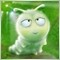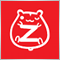# TextCreate 在 EA里 不起作用 么53

```bool TextCreate(const long              chart_ID=0,               // 图表 ID
const string            name="Text",              // 对象名称
const int               sub_window=0,             // 子窗口指数
datetime                time=0,                   // 定位点时间
double                  price=0,                  // 定位点价格
const string            text="Text",              // 文本本身
const string            font="Arial",             // 字体
const int               font_size=10,             // 字体大小
const color             clr=clrRed,               // 颜色
const double            angle=0.0,                // 文本倾斜
const ENUM_ANCHOR_POINT anchor=ANCHOR_LEFT_UPPER, // 定位类型
const bool              back=false,               // 在背景中
const bool              selection=false,          // 突出移动
const bool              hidden=true,              // 隐藏在对象列表
const long              z_order=0)                // 鼠标单击优先
{
//--- 若未设置则设置定位点的坐标
ChangeTextEmptyPoint(time,price);
//--- 重置错误的值
ResetLastError();
//--- 创建文本对象
if(!ObjectCreate(chart_ID,name,OBJ_TEXT,sub_window,time,price))
{
Print(__FUNCTION__,
": failed to create \"Text\" object! Error code = ",GetLastError());
return(false);
}
//--- 设置文本
ObjectSetString(chart_ID,name,OBJPROP_TEXT,text);
//--- 设置文本字体
ObjectSetString(chart_ID,name,OBJPROP_FONT,font);
//--- 设置字体大小
ObjectSetInteger(chart_ID,name,OBJPROP_FONTSIZE,font_size);
//--- 设置文本的倾斜角
ObjectSetDouble(chart_ID,name,OBJPROP_ANGLE,angle);
//--- 设置定位类型
ObjectSetInteger(chart_ID,name,OBJPROP_ANCHOR,anchor);
//--- 设置颜色
ObjectSetInteger(chart_ID,name,OBJPROP_COLOR,clr);
//--- 显示前景 (false) 或背景 (true)
ObjectSetInteger(chart_ID,name,OBJPROP_BACK,back);
//--- 启用 (true) 或禁用 (false) 通过鼠标移动对象的模式
ObjectSetInteger(chart_ID,name,OBJPROP_SELECTABLE,selection);
ObjectSetInteger(chart_ID,name,OBJPROP_SELECTED,selection);
//--- 在对象列表隐藏(true) 或显示 (false) 图形对象名称
ObjectSetInteger(chart_ID,name,OBJPROP_HIDDEN,hidden);
//--- 设置在图表中优先接收鼠标点击事件
ObjectSetInteger(chart_ID,name,OBJPROP_ZORDER,z_order);
//--- 成功执行
return(true);
}
//+------------------------------------------------------------------+
//| 检查定位点的值和为空点设置                                           |
//| 默认的值                                                          |
//+------------------------------------------------------------------+
void ChangeTextEmptyPoint(datetime &time,double &price)
{
//--- 如果点的时间没有设置，它将位于当前柱
if(!time)
time=TimeCurrent();
//--- 如果点的价格没有设置，则它将用卖价值
if(!price)
price=SymbolInfoDouble(Symbol(),SYMBOL_BID);
}
//+------------------------------------------------------------------+
```

在 EA 的   OnTick(void)   部分  加入代码        TextCreate(0,"TextHigh_",0,iTime(NULL,0,0),iHigh(NULL,0,0)," rrr  ","Arial",20,
clrRed,90.0,ANCHOR_LEFT,false,false,true,0);   但不会看到 显示 的文字  同样 的   代码   函数  放到   脚本   或  指标 里  就 可以显示 文字 ，

EA 里  不可以 用 该类 文本 显示 类 函数 么 ？14746

jldfj:

在 EA 的   OnTick(void)   部分  加入代码        TextCreate(0,"TextHigh_",0,iTime(NULL,0,0),iHigh(NULL,0,0)," rrr  ","Arial",20,
clrRed,90.0,ANCHOR_LEFT,false,false,true,0);   但不会看到 显示 的文字  同样 的   代码   函数  放到   脚本   或  指标 里  就 可以显示 文字 ，

EA 里  不可以 用 该类 文本 显示 类 函数 么 ？53

Jinsong Zhang:

EA 回测期间 也不起作用么30736